# Phased Array Antenna Patterns—Part 1: Linear Array Beam Characteristics and Array Factor

### Introduction

With the proliferation of digital phased arrays in commercial and aerospace and defense applications, there are many engineers working on various aspects of the design who have limited phased array antenna familiarity. Phased array antenna design is not new, as the theory has been well developed over decades; however, most of the literature is intended for antenna engineers well versed in the electromagnetic mathematics. As phased arrays begin to include more mixed-signal and digital content, there are many engineers who could benefit from a much more intuitive explanation of phased array antenna patterns. As it turns out, there are many analogies between the behavior of phased array antennas and the discrete time sampled systems that the mixed-signal and digital engineers work with every day.

These articles are not intended to create antenna design engineers, but rather to help the engineer working on a subsystem or component used in a phased array to visualize how their effort may impact a phased array antenna pattern.

### Beam Direction

First, let’s look at an intuitive example of steering a phased array beam. Figure 1 provides a simple illustration of a wavefront striking four antenna elements from two different directions. A time delay is applied in the receive path after each antenna element, and then all four signals are summed together. In Figure 1a, that time delay matches the time difference of the wavefront striking each element. And in this case, that applied delay causes the four signals to arrive in phase at the point of combination. This coherent combining results in a larger signal at the output of the combiner. In Figure 1b, that same delay is applied; however, in this case, the wavefront is perpendicular to the antenna elements. That applied delay now misaligns the phase of the four signals, and the output of the combiner is significantly reduced.

In a phased array, time delay is the quantifiable delta needed for beam steering. But time delay can also be emulated with a phase shift, which is common and practical in many implementations. We will discuss the impact of time delay vs. phase shift in the section on beam squint, but for now let’s look at a phase shift implementation, and then derive the calculation for beam steering with that phase shift.

Figure 2 shows this phased array arrangement using phase shifters rather than time delay. Note that we define the boresight direction (θ = 0º) as perpendicular to the face of the antenna. A positive angle θ is defined to the right of boresight, and a negative angle is defined to the left of boresight.

To visualize the phase shift needed for beam steering, a set of right triangles can be drawn between adjacent elements, as shown in Figure 3. Where ΔΦ is the phase shift between those adjacent elements.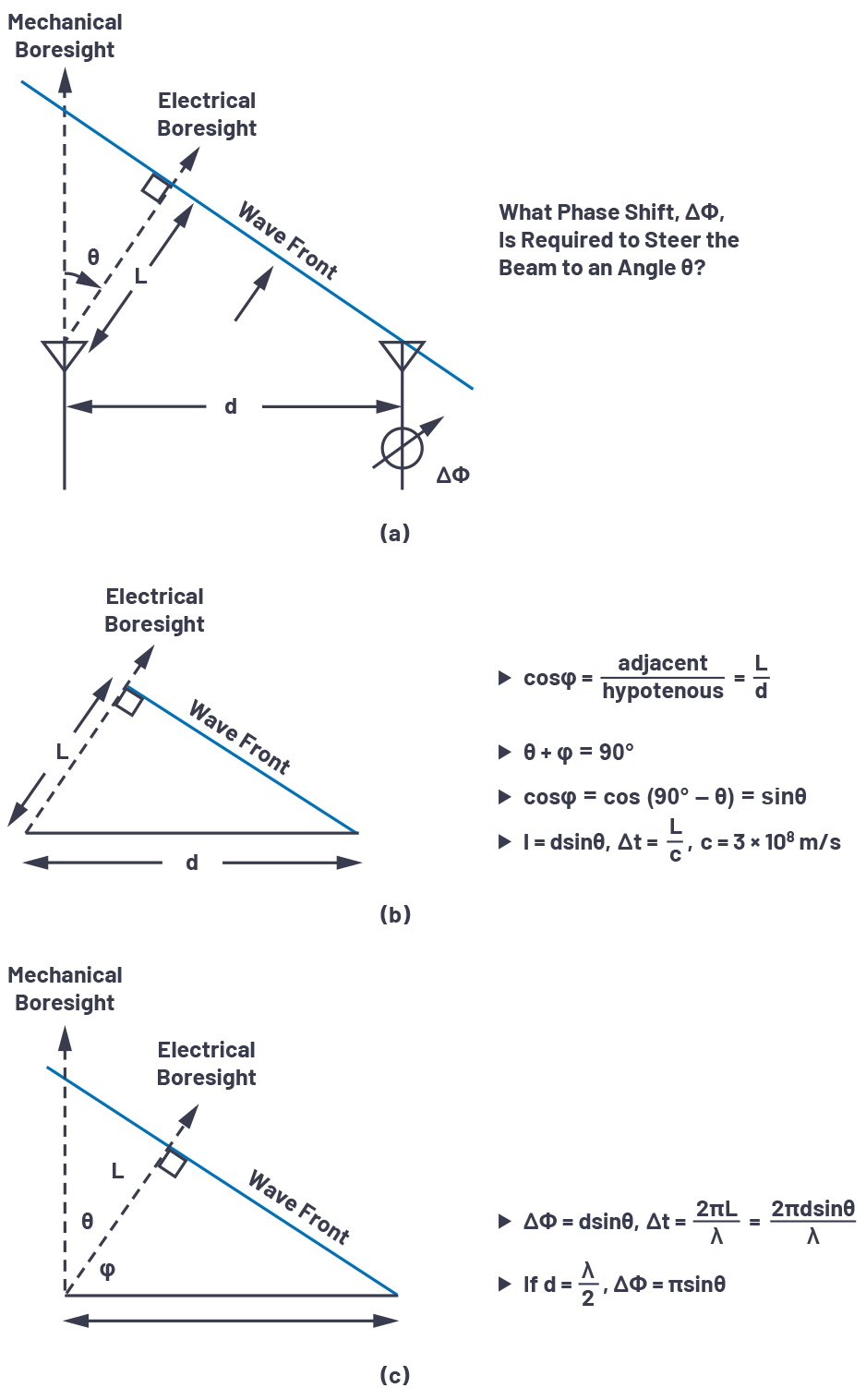Figure 3. Derivation of phase shift ΔΦ vs. beam steering angle.

Figure 3a defines the trigonometry between those elements, with each element separated by a distance (d). The beam is pointed in a direction off boresight, θ, which is an angle, φ, from the horizon. In Figure 3b, we see that the sum of θ + φ = 90o. This allows us to compute L, the delta distance of wave propagation, as L = dsin(θ). The time delay to steer our beam is equal to the time it will take for the wavefront to traverse that distance, L. If we think of L as a fraction of the wavelength, then a phase delay could be substituted in for that time delay. The equations for ΔΦ can then be defined relative to θ, as shown in Figure 3c and repeated in Equation 1.

If the spacing between elements is exactly one half of the signal wavelength, then this can further be simplified to:

Let’s work out an example with these equations. Consider two antenna elements spaced 15 mm apart. If a 10.6 GHz wavefront is arriving at 30º from mechanical boresight, then what is the optimal phase shift between the two elements?

• θ = 30º = 0.52 rad
• λ = c/f = (3 × 108 m/s)/10.6 GHz = 0.0283 m
• ∆Φ = (2π × d × sinθ)/λ = 2π × 0.015 × sin(0.52)/0.0283 m = 1.67 rad = 95º

So, if our wavefront is arriving at θ = 30º, then if we shift the phase of the neighboring element by 95º, we will cause the individual signals of both elements to add coherently. This will maximize the antenna gain in that direction.

For a better appreciation of how the phase shift varies with the beam direction (θ), these equations are plotted for a variety of conditions in Figure 4. Some interesting observations can be made from these graphs. For the case of d = λ/2, there is an approximate 3 to 1 slope near boresight, which is the π multiplier in Equation 2. This case also shows a full 180° shift between elements provides a theoretical 90° shift in beam direction. In practice, with real element patterns, this is not realizable, yet the equations do show the theoretical ideal. Note that for d > λ/2, no amount of phase shift provides a full beam shift. Later, we will see this case can lead to grating lobes in the antenna pattern, and this graph provides a first indicator that something is different with the d > λ/2 case.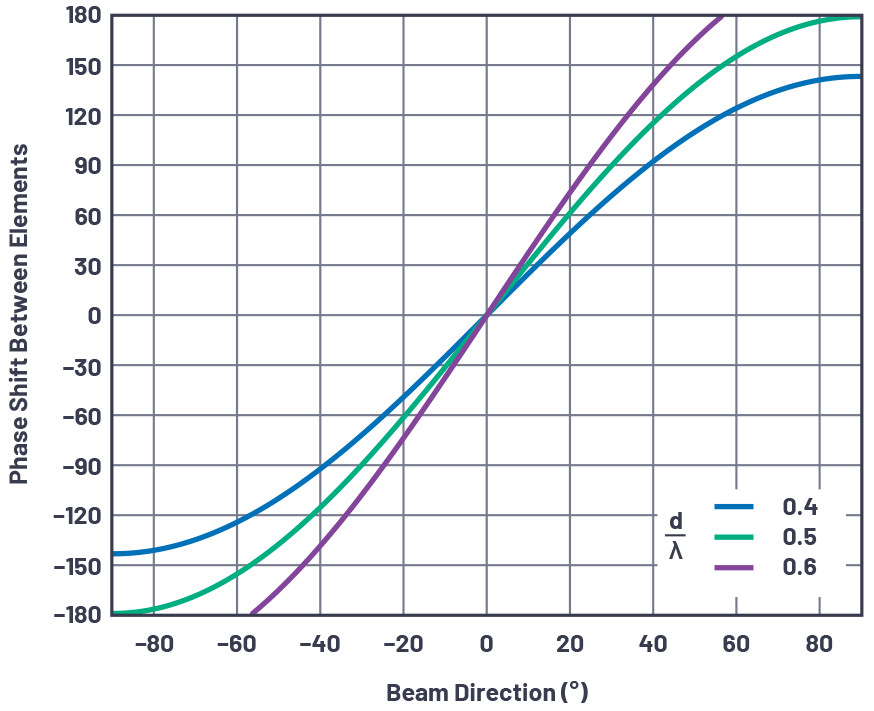Figure 4. Phase shift ΔΦ between elements vs. beam direction (θ) for three cases of d/λ.

### A Uniformly Spaced Linear Array

The equations developed above have applied to just two elements. Yet a real phased array can be thousands of elements spaced across two dimensions. But for our purposes here, let’s just consider one dimension: a linear array.

A linear array is a single element wide with N number of elements across. The spacing may vary, but often it is uniform. Therefore, in this paper, we will set the spacing between each element to a uniform distance, d, (Figure 5). Although simplified, this uniformly spaced linear array model provides the foundation for insight into how the antenna pattern is formed vs. a variety of conditions. We can further apply the principles of the linear array to understand two-dimensional arrays.Figure 5. A uniformly spaced linear array (N = 4).

### Near Field vs. Far Field

So how can we take the equations previously developed for an N = 2 linear array and apply them to an N = 10,000 linear array? Right now, it seems that each antenna element has a slightly different angle pointing to the spherical wavefront, shown in Figure 6.

With the RF source near, the incident angle varies for each element. This situation is called the near field. We can work out all these angles, and sometimes we need to do this for antenna testing and calibration as our test setup can only be so large. But, if we instead just assumed that the RF source was far away, then we’d have the case in Figure 7.Figure 7. An RF source far from the linear array.

With the RF source far away, the large radius of the spherical wavefront results in wave propagation paths that are approximately parallel. Therefore, all our beam angles are equal, and each adjacent element has a path length that is L = d × sinθ longer than its neighbor. This simplifies the math and means that the two element equations we derived can be applied to thousands of elements, provided they have uniform spacing.

But when can we make the far field assumption? How far is far? It’s a little subjective, but in general, far field is considered anything greater than:

where D is the diameter of the antenna ((N-1) × d for our uniform linear array)

For a small array (small D) or a low frequency (large λ), the far field distance is small. But for a large array (or high frequency), the far field distance could be many kilometers! That makes it hard to test and calibrate the array. For those conditions, a more detailed near model may be used, and then bridge this back to the far field, real-world use of the array.

### Antenna Gain, Directivity, and Aperture

Before we go too far, it is helpful to define antenna gain, directivity, and aperture. Let’s start with a clarification on gain vs. directivity, as the two are often interchanged. Antenna gain and directivity are in comparison to an isotropic antenna—which is an ideal antenna that radiates evenly in all directions. Directivity is a comparison of the maximum power measured, Pmax, in a particular direction to the average power radiated across all directions, Pav. When no direction is defined, directivity is determined by Equation 4.

Directivity is a useful metric when comparing antennas as it defines the ability to focus radiated energy. Gain has the same pattern of directivity, but gain includes the antenna losses.

Prad is the total power radiated, Pin is the input power to the antenna, and k accounts for losses in the antenna radiation process.

Next, let’s consider an antenna pattern as a function of a three-dimensional direction and directivity as a function of beamwidth.Figure 8. A three-dimensional view of an area projected onto a sphere.

The total surface area of a sphere is 4π2, and an area on a sphere is defined in units of steradians with 4π steradians in a sphere. Therefore, the power density from an isotropic radiator is

and has units of (W/m2).

There are two angular directions for an area of a sphere. In radar systems, these are commonly referred to as azimuth and elevation. Beamwidths can be described as a function of each angular direction as θ1 and θ2: the combination creates an area on the sphere of ΩA.

ΩA is the beamwidth in steradians and can be approximated as ΩA ≈ θ1 × θ2.

Recognizing ΩA as an area on the sphere, directivity can then be expressed as

The third antenna term we’ll consider is aperture. Antenna aperture represents an effective area for receiving electromagnetic waves and includes a function relative to wavelength. The aperture of an isotropic antenna is

Gain is relative to isotropic radiation, making the effective aperture of an antenna

Pulling these three terms together, we can see that gain can be considered a function of angle that defines a radiation pattern and accounts for efficiency (or losses) in the antenna.

### Array Factor for a Linear Array

At this point, we’re able to predict the optimal time (or phase) delta between elements to achieve maximum antenna directivity. But we’d really like to understand and manipulate the complete antenna gain pattern. There are two main parts to this. First, there is the gain of each individual element of our array (perhaps one patch), called the element factor (GE). Secondly, there is the impact that we can exert through beamforming the array, called the array factor (GA). The full array antenna gain pattern is the combination of the two factors, as shown in Equation 10.

The element factor, GE, is the radiating pattern of a single element in the array. This is defined by the geometry and construction of the antenna, and not something that is varied in operation. It is important to know, as it will limit the gain of our total array—particularly near the horizon. But since we can’t control it electrically, we’ll leave it as a fixed influencer to our total phased array gain equation. For this article, we’ll assume that all the individual elements have the same element factor.

The focus then is on the array factor, GA. The array factor is calculated based on array geometry (d for our uniform linear array) and beam weights (amplitude and phase). Deriving the array factor for a uniform linear array is straightforward, but the details are best covered in the references cited at the end of this article.

There are some variations in equations used across literature depending on how parameters were defined in the linear array. We use the equations from this article, which results in consistency with our definitions in Figure 2 and Figure 3. Since our primary concern is how the gain changes, it is often more instructive to plot the normalized array factor relative to unity gain. That normalized array factor can be written as Equation 11.

We have already defined beam angle θ0 as a function of phase shift between elements ΔΦ; therefore, we can also write the normalized antenna factor as Equation 12.

The conditions assumed in the array factor equation include:

• The elements are equally spaced.
• There is an equal phase shift between elements.
• The elements are all at equal amplitude.

Next, using these equations, we plot the array factor for several array sizes.Figure 10. Normalized array factor at boresight of a linear array with an element spacing of d = λ/2 and an element count of 8, 16, and 32.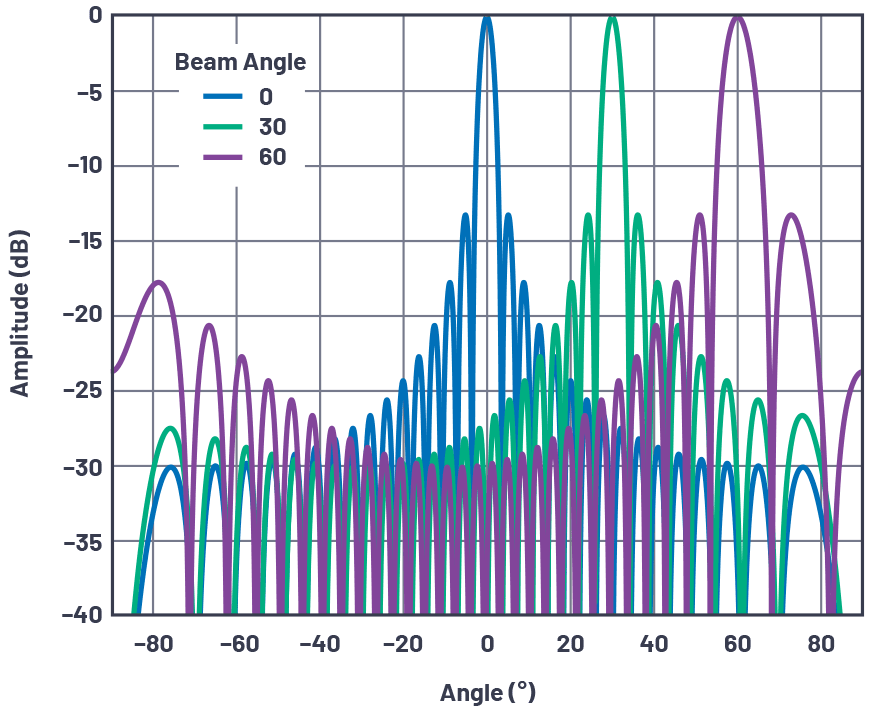Figure 11. Normalized array factor of a 32-element linear array at several beam angles with an element spacing of d = λ/2.

Some observations from these figures include:

• The first sidelobe is at –13 dBc regardless of the element count. This is due to the sinc function in the array factor equation. The sidelobes can be improved with tapering the gain across elements and will be the subject of an upcoming section in this series.
• The beamwidth reduces with the number of elements.
• The beamwidth widens as the beam is scanned away from boresight.
• The number of nulls increases as the number of elements increases.

### Beamwidth

Beamwidth provides a metric of angular resolution for antennas. Most commonly, beamwidth is defined by either the half-power beamwidth (HPBW) or the null-to-null spacing of the main lobe (FNBW). To find the HPBW, we move 3 dB down from the peak and measure the angular distance, as shown in Figure 12.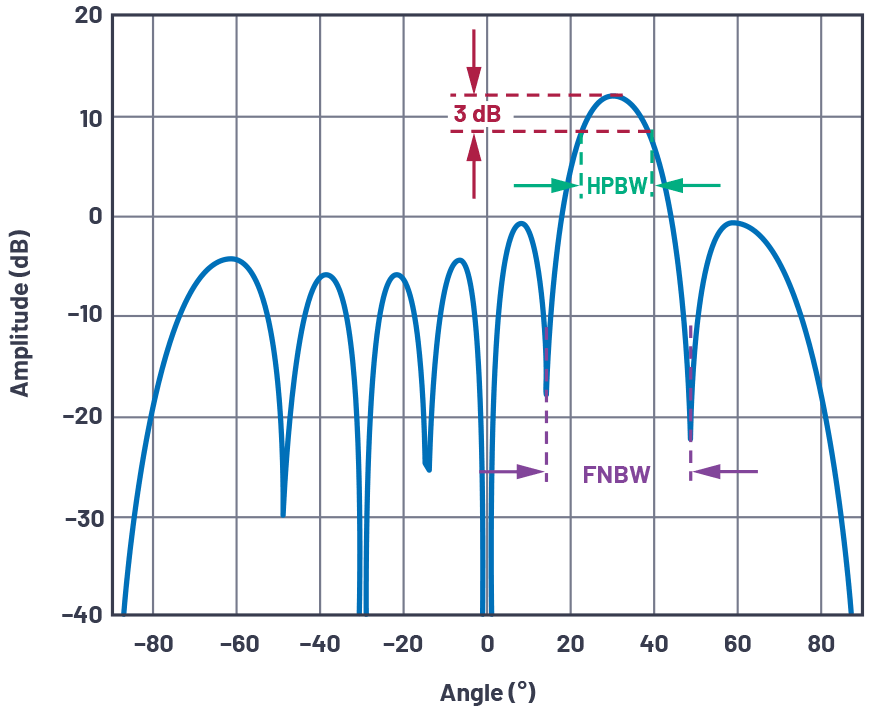Figure 12. Definition of antenna beamwidth (linear array of N = 8, d = λ/2, θ = 30° shown).

Using our normalized array factor equation, we can solve for this HPBW by setting Equation 3 equal to the half-power level (3 dB or 1/√ 2). We’ll assume mechanical boresight (θ = 0º), N = 8, and d = λ/2.

Then solving for ∆Φ gives 0.35 rad. Use Equation 1, and solve for θ:

That θ is the peak to 3 dB point, which is half of our HPBW. Therefore, we simply double it to arrive at the angular distance between the 3 dB points. This results in an HPBW of 12.8º.

We could repeat this for an array factor equal to 0 and obtain the first null-to-null spacing angle of FNBW = 28.5º, for the previously mentioned conditions.

For uniform linear arrays, an approximation for HPBW [1,2] is given as Equation 15.

Figure 13 plots beamwidth vs. beam angle for several element counts in the condition of a λ/2 element spacing.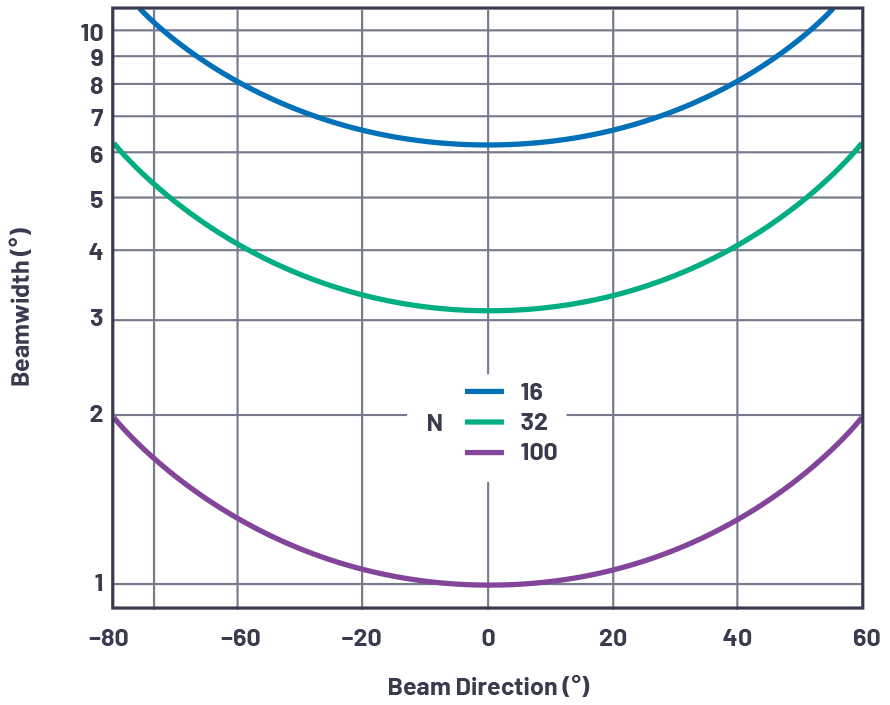Figure 13. Beamwidth vs. beam angle at an element spacing of λ/2 for an element count of 16, 32, and 100 elements.

From this graph, it is worth noting some observations relative to array sizes under development in the industry.

• A 1° beam accuracy requires 100 elements. If this is desired in both azimuth and elevation, this results in a 10,000 element array. The 1° accuracy is only at boresight under near ideal conditions. Maintaining 1° accuracy in a fielded array across a variety of scan angles will increase the element count further. This observation then sets a practical limit for beamwidth with very large arrays.
• A 1000-element array is common in the industry. 32 elements in each direction provides an element count of 1024, and can yield a beam accuracy less than 4° near boresight.
• A 256-element array, which can be mass produced at low cost, can still have a beam pointing accuracy less than 10°. This may be perfectly acceptable for many applications.
• Also note that for any of these cases, the beamwidth doubles at 60° offsets. This is from the cosθ in the denominator and is due to the foreshortening of the array; that is, the array appears to be a smaller cross section when viewed from an angle.

### Combining Element and Array Factors

The previous section only considered the array factor. But to find the total antenna gain, we also require the element factor. Figure 14 illustrates an example. In this example, we use a simple cosine shape as the element factor, or normalized element gain, GE(θ). The cosine rolloff is common in phased array analysis and can be visualized if considering a flat surface. At broadside, there is a maximum area. As the angle moves away from broadside, the area visible reduces following a cosine function.

The array factor, GA(θ), was used for a 16-element linear array, with a λ/2 spacing, and a uniform radiation pattern. The total pattern is a linear multiplication of the element factor and array factor, so in a dB scale, they can be added together.Figure 14. Element factor and array factor combine to form the total antenna pattern.

A few observations as the beam moves off boresight:

• The main beam loses amplitude at the rate of the element factor.
• The sidelobes on boresight have no amplitude loss.
• The result is the sidelobe performance of the overall array degraded off boresight.

### Antenna Plots: Cartesian vs. Polar

The antenna pattern plots used so far have been in cartesian coordinates. But it is common to plot antenna patterns in polar coordinates as they are more representative of energy radiating spatially outwards from the antenna. Figure 15 is a redrawn version of Figure 12, but using polar coordinates. Note that this is the exact same data, point for point—it’s just redrawn with a polar coordinate system. It is worthwhile to be able to visualize the antenna pattern in either representation as both are used in literature. For most of this text, we will use cartesian coordinates as in this representation it can be easier to compare beamwidth and sidelobe performance.Figure 15. Polar coordinate antenna directivity plot for N = 8, d = λ/2, θ = 30°.

### Array Reciprocity

Up until this point, all the diagrams and text have described a signal that the array is receiving. But how would this change for a transmit array? Fortunately, most antenna arrays are reciprocal. Therefore all of the diagrams, equations, and terminology are the same for transmit as they are for receive. Sometimes it is easier to think of the beam as being received by the array. And sometimes, perhaps in the case of grating lobes, you may find it more intuitive to think of the array as transmitting a beam. In this article, we generally describe the array as receiving a signal. But if this is harder to visualize for you, then you can equally think of the same concepts on the transmit side.

### Summary

This concludes Part 1 of the series. The concept of beam steering with a phased array was introduced. The equations to calculate phase shift across the array for beam steering were derived and shown graphically. Then array factor and element factor were defined with observations of how the number of elements, the spacing between elements, and the beam angle impacts the antenna response. Finally, a comparison of antenna patterns in cartesian vs. polar coordinates was shown.

Upcoming articles in this series will further explore phased array antenna patterns and impairments. We’ll study how antenna tapering reduces sidelobes, how grating lobes are formed, and the impact of phase shift vs. time delay in wideband systems. The series will finish with an analysis of the finite resolution of the delay block and how it can create quantization sidelobes and degrade beam resolution.

### Ссылки

Balanis, Constantine A. Antenna Theory: Analysis and Design. Third edition. Wiley, 2005.

Mailloux, Robert J. Phased Array Antenna Handbook. Second edition, Artech House, 2005.

O’Donnell, Robert M. “Radar Systems Engineering: Introduction.” IEEE, June 2012.

Skolnik, Merrill. Radar Handbook. Third edition, McGraw-Hill, 2008.